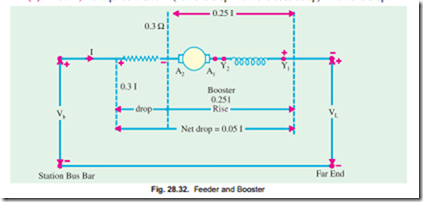# Generator characteristic:Compound-wound Generator

### Compound-wound Generator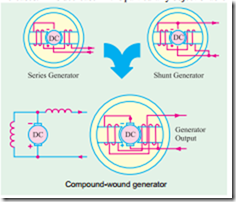A shunt generator is unsuitable where constancy of terminal voltage is essential, because its terminal voltage decreases as the load on it increases. This decrease in V is particularly objectionable for lighting circuit where even slight change in the voltage makes an appreciable change in the candle power of the incandescent lamps. A shunt generator may be made to supply substantially constant voltage (or even a rise in voltage as the load increases) by adding to it a few turns joined in series with either the armature or the load (Fig. 28.24). These turns are so connected as to aid to shunt turns when the generator supplies load. As the load current increases, the current through the series windings also increase thereby increasing the flux. Due to the increase in flux, induced e.m.f. is also increased. By adjusting the number of series turns (or Compound-wound generator series amp-turns), this increase in e.m.f. can be made to balance the combined voltage drop in the generator due to armature reaction and the armature drop. Hence, V remains practically constant which means that field current is also almost unchanged. We have already discussed the three causes which decrease the terminal voltage of a shunt generator (Art 28.10). Out of these three, the first two are neutralized by the series field amp-turns and the third one, therefore, does not occur.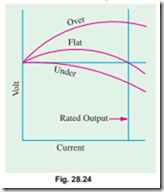If the series field amp-turns are such as to produce the same voltage at rated load as at no-load, then the generator is flat-compounded. It should be noted, however, that even in the case of a flat-compounded genera- tor, the voltage is not constant from no-load to rated- load. At half the load, the voltage is actually greater than the rated voltage as seen from Fig. 28.24.

If the series field amp-turns are such that the rated-load voltage is greater than the no-load voltage, then generator is over- compounded. If rated-load voltage is less than the no-load voltage, then the generator is under-compounded but such generators are seldom used.

For short distances such as in hotels and office buildings, flat-compound generators are used because the loss of voltage over small lengths of the feeder is negligible. But when it is necessary to maintain a constant voltage then an over- compounded generator, which combines the functions of a Fig. 28.24 generator and a booster, is invariably used.

### How to Calculate Required Series Turns ?

Consider a 110-V, 250-ampere generator. Suppose it gives its rated no-load voltage with a field current of 5.8 A. If, now, the series windings are disconnected and the shunt field rheostat is left unchanged then the machine will act as shunt generator, hence its voltage will fall with increase in load current. Further, supply that the field current has to be increased to 6.3 A in order to maintain the rated terminal voltage at full load. If the number of turns of the shunt field winding is 2000, then 2000 ´ (6.3 – 5.8) = 1000 amp-turns represent the additional excitation that has to be supplied by the series windings. As series turns will be carrying a full load current of 250 A, hence number of series turns = 1000/250 = 4.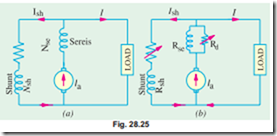In general, let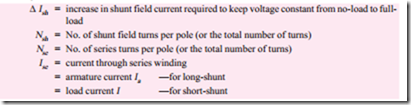It is seen that while running as a simple generator, the increase in shunt field ampere-turns necessary for keeping its voltage constant from no-load to full-load is Nsh · Dsh. This increase in field excitation can be alternatively achieved by adding a few series turns to the shunt generator [Fig. 28.25 (a)] thereby converting it into a compound generator.

Nsh · D Ish = Nse Ise

If other things are known, Nse may be found from the above equation.

In practice, a few extra series amp-turns are taken in order to allow for the drop in armature. Any surplus amp-turns can be changed with the help of a divertor across the series winding as shown in Fig. 28.25 (b).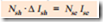As said above, the degree of compounding can be adjusted with the help of a variable-resistance, divertor as shown in Fig. 28.25 (b). If Id is the current through the divertor of resistance Rd, then remembering that series windings and divertor are in parallel,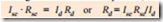Example 28.15. A shunt generator is converted into a compound generator by addition of a series field winding. From the test on the machine with shunt excitation only, it is found that a field current of 5 A gives 440 V on no-load and that 6 A gives 440 V at full load current of 200 A. The shunt winding has 1600 turns per pole. Find the number of series turns required.

(Elect. Machines, A.M.I.E., Sec., B, 1991) Solution. It would be assumed that shunt generator is converted into a short shunt compound generator. It is given that for keeping the voltage of shunt generator constant at 440 V both at no load and full-load, shunt field ampere-turns per pole have to be increased from 1600 ´ 5 = 8000 to (1600 ´ 6) = 9600 i.e. an increase of (9600 – 8000) = 1600 AT. The same increase in field AT can be brought about by adding a few series turns.

Let n be the number of series turns required per pole. Since they carry 200 A,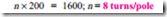Example 28.16. A long shunt compound generator has a shunt field winding of 1000 turns per pole and series field winding of 4 turns per pole and resistance 0.05 W. In order to obtain the rated voltage both at no-load and full-load for operation as shunt generator, it is necessary to increase field current by 0.2 A. The full-load armature current of the compound generator is 80 A. Calculate the divertor resistance connected in parallel with series field to obtain flat compound operation.

#### (Elect. Machines A.M.I.E. Sec. B, 1993)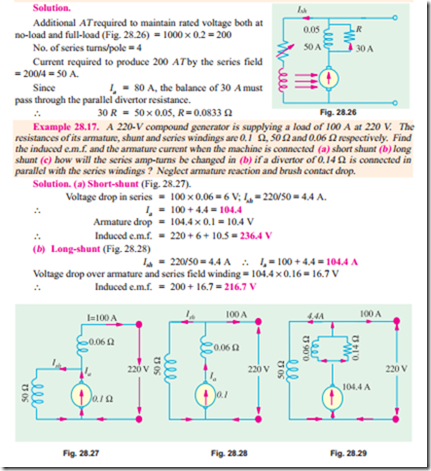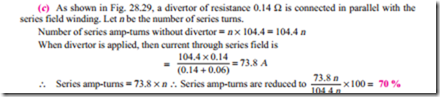Example 28.18. A 250-kW, 240-V generator is to be compounded such that its voltage rises from 220 volts at no-load to 240 V at full load. When series field is cut out and shunt field is excited from an external source, then from the load test it is found that this rise in voltage can be obtained by increasing the exciting current from 7 A at no-load to 12 A at full-load. Given shunt turns/pole = 650, series turns/pole = 4 and resistance of series winding, 0.006 W. If the machine is connected long-shunt, find the resistance of the series amp-turns at no-load and drop in series winding resistance at full-load.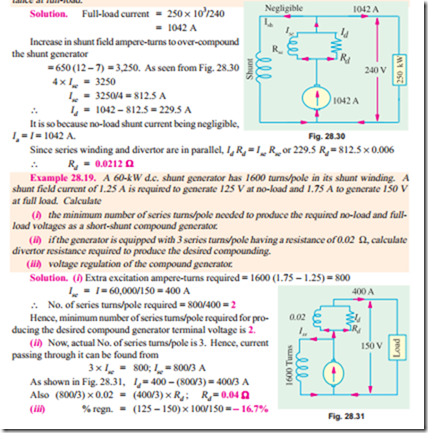Example 28.20. A D.C. generator having an external characteristics which is a straight line through zero to 50 V at 200 Amp, is connected as a booster between a station bus-bar and a feeder of 0.3 ohm resistance. Calculate the voltage between the far end of the feeder and the bus-bar at a current of (i) 160 A, (ii) 50 A. (Manomaniam Surdaranar Univ. Nov. 1998) Solution. Due to the feeder resistance of 0.3 ohm there is a voltage drop of 1 ´ 0.3 volts in the direction of current, where I refers to the current flowing. Due to booster, there is a rise in voltage given by I ´ (50/200) according to the VI characteristic given for the series generator. As a sum total of these two, the net feeder drop will be I ´ (0.3 – 0.25) or I volts, and that represents the voltage between far end of the feeder and the bus-bar.

(i) At 160 A, the net voltage drop in feeder = 160 ´ 0.05 or 48 – 40 = 9 volts.

Since 48 volts drop is partially compensated by 40 volts boosted up.

(ii) At 50 A, the required answer = (15 volts drop 12.5 volts boosted up) = 2.5 volts drop.